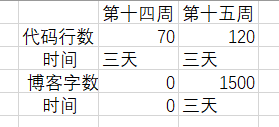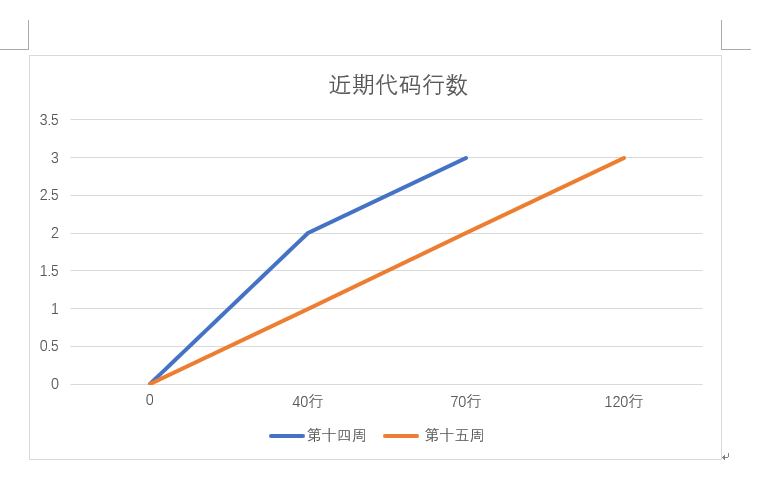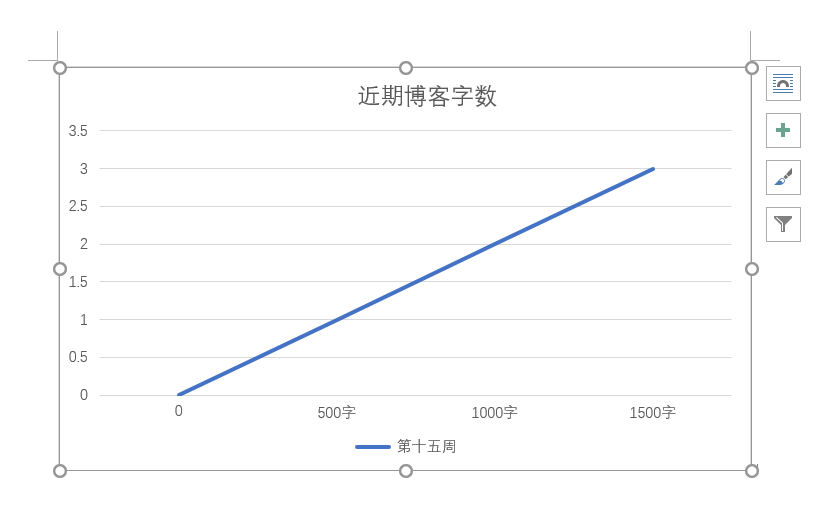### 题目7-1 判断素数

1.实验代码

#include<stdio.h>
#include<math.h>
int main(void)

{
int x,a,n,b;
scanf("%d",&n);
while(n--){
scanf("%d",&x);
b=1;
for(a=2;a<=sqrt(x);a++){
if(x%a==0){
b=0;
break;
}
}
if(b==0||x==1)
printf("No\n");
else
printf("Yes\n");
}
return 0;
}

2 设计思路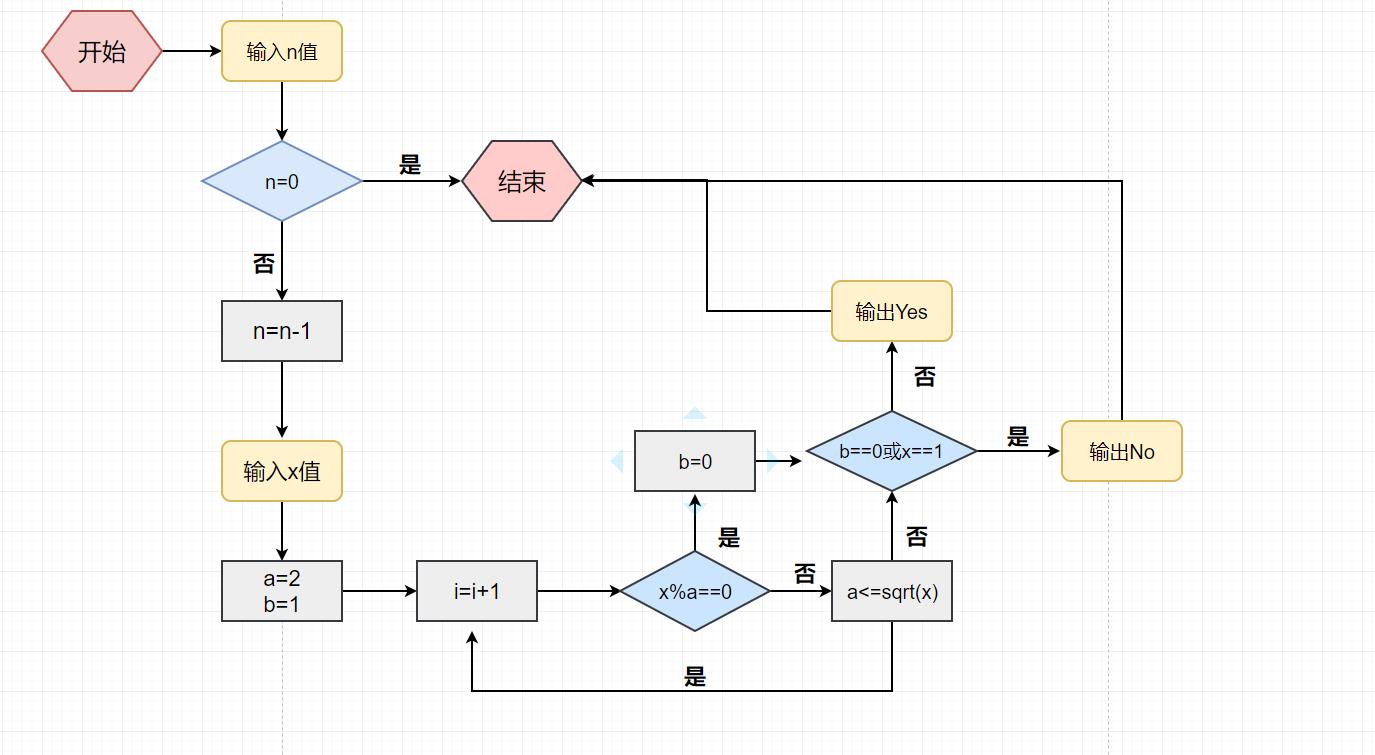#### 3.本题调试过程碰到问题及解决办法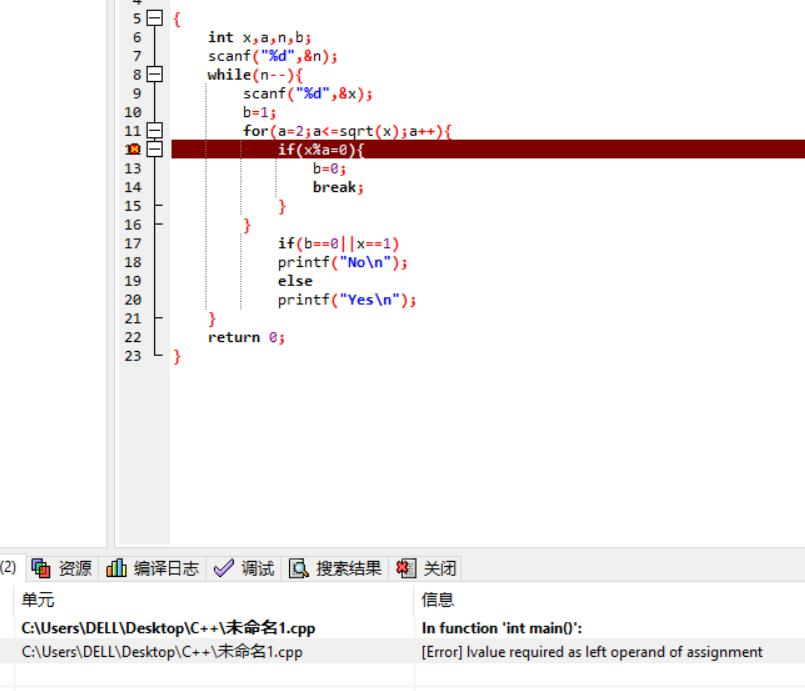'=='  少打了一个 '='

#### 1.实验代码

#include<stdio.h>
int main(){
char c,s;
int i,a=0,b=0;
gets(s);
for(i=0;;i++)
{
c = s[i];
if(c=='\0'){
break;　　　　　　　　　　}
if(c==' '){　　　　　　b=0;　　　　　　　　　　}
else if(b == 0)
{
b = 1;
a++;
}
}
printf("%d",a);

return 0;
}

#### 2 设计思路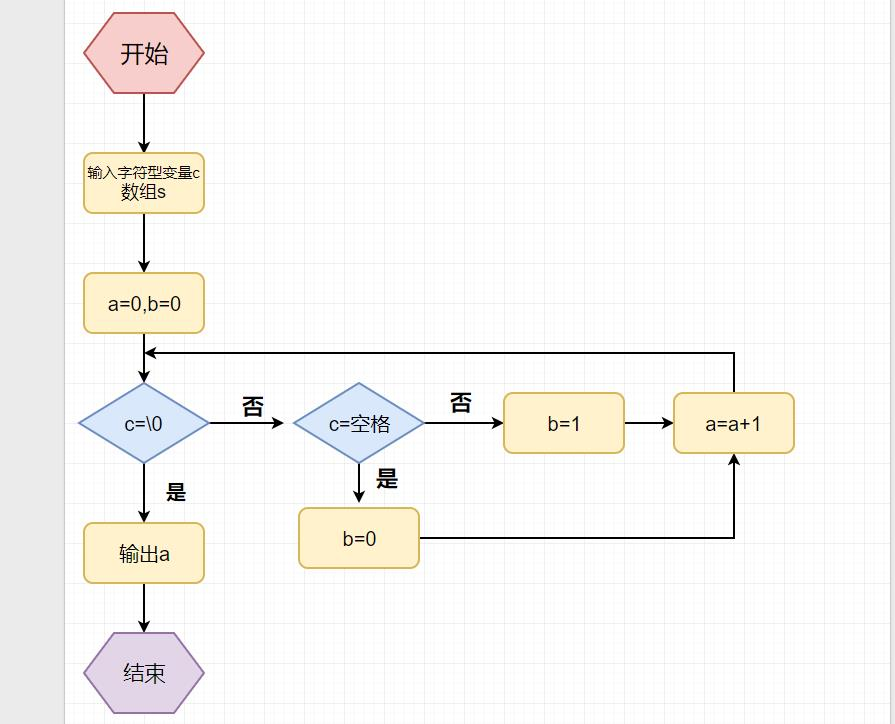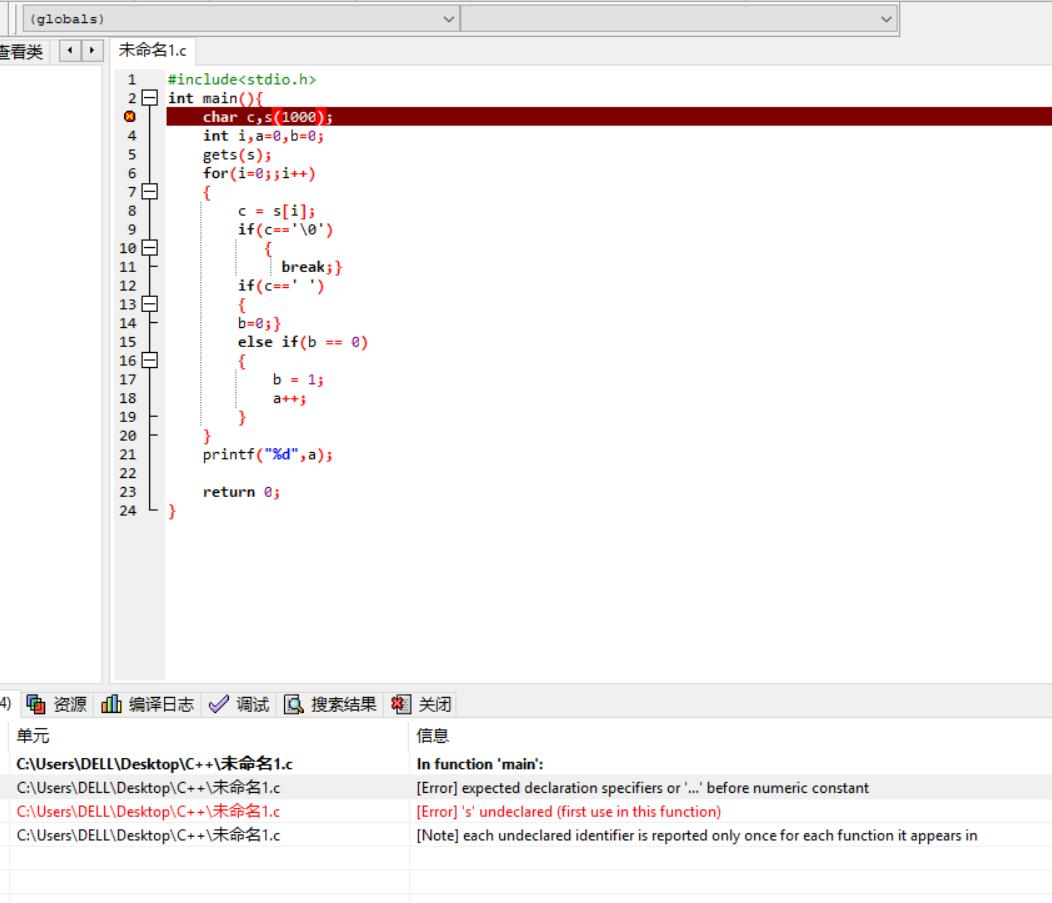1、总结这几周所学内容

2、点评3个同学的本周作业

3、请用表格和折线图呈现你这几周的代码行数和时间、博客字数和时间、涉及的知识点。